# OSCILLATIONS AND WAVES - MECHANICAL OSCILLATIONS

MECHANICAL OSCILLATIONS

1. Harmonic motion equation and its solution: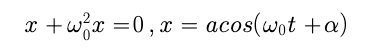where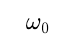is the natural oscillation frequency

2. Damped oscillation equation and its solution: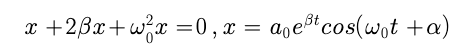where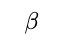is the damping coefficient,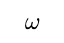is the frequency of damped oscillation;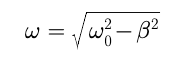3.  Logarithmic damping decrement ? and quality factor Q: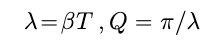where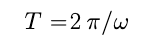4.Forced oscillation equation and its steady-state solution: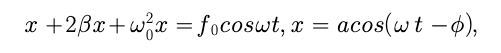Where5.Maximum shift amplitude occurs at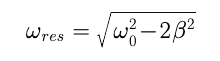Post By : Faiz Ahmad Faiz 24 Apr, 2020 263 views Physics